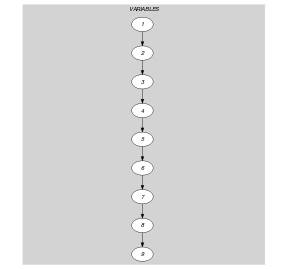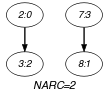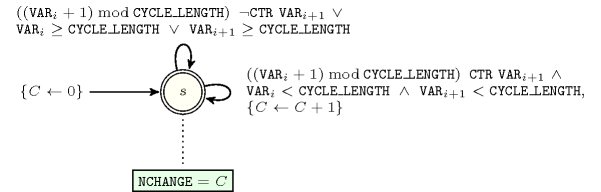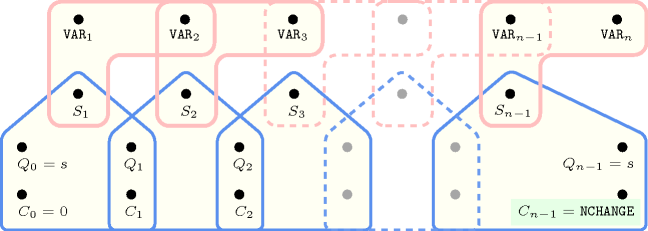## 5.108. cyclic_change_joker

Origin
Constraint

$\mathrm{𝚌𝚢𝚌𝚕𝚒𝚌}_\mathrm{𝚌𝚑𝚊𝚗𝚐𝚎}_\mathrm{𝚓𝚘𝚔𝚎𝚛}\left(\mathrm{𝙽𝙲𝙷𝙰𝙽𝙶𝙴},\mathrm{𝙲𝚈𝙲𝙻𝙴}_\mathrm{𝙻𝙴𝙽𝙶𝚃𝙷},\mathrm{𝚅𝙰𝚁𝙸𝙰𝙱𝙻𝙴𝚂},\mathrm{𝙲𝚃𝚁}\right)$

Arguments
 $\mathrm{𝙽𝙲𝙷𝙰𝙽𝙶𝙴}$ $\mathrm{𝚍𝚟𝚊𝚛}$ $\mathrm{𝙲𝚈𝙲𝙻𝙴}_\mathrm{𝙻𝙴𝙽𝙶𝚃𝙷}$ $\mathrm{𝚒𝚗𝚝}$ $\mathrm{𝚅𝙰𝚁𝙸𝙰𝙱𝙻𝙴𝚂}$ $\mathrm{𝚌𝚘𝚕𝚕𝚎𝚌𝚝𝚒𝚘𝚗}\left(\mathrm{𝚟𝚊𝚛}-\mathrm{𝚍𝚟𝚊𝚛}\right)$ $\mathrm{𝙲𝚃𝚁}$ $\mathrm{𝚊𝚝𝚘𝚖}$
Restrictions
 $\mathrm{𝙽𝙲𝙷𝙰𝙽𝙶𝙴}\ge 0$ $\mathrm{𝙽𝙲𝙷𝙰𝙽𝙶𝙴}<|\mathrm{𝚅𝙰𝚁𝙸𝙰𝙱𝙻𝙴𝚂}|$ $\mathrm{𝙲𝚈𝙲𝙻𝙴}_\mathrm{𝙻𝙴𝙽𝙶𝚃𝙷}>0$ $\mathrm{𝚛𝚎𝚚𝚞𝚒𝚛𝚎𝚍}$$\left(\mathrm{𝚅𝙰𝚁𝙸𝙰𝙱𝙻𝙴𝚂},\mathrm{𝚟𝚊𝚛}\right)$ $\mathrm{𝚅𝙰𝚁𝙸𝙰𝙱𝙻𝙴𝚂}.\mathrm{𝚟𝚊𝚛}\ge 0$ $\mathrm{𝙲𝚃𝚁}\in \left[=,\ne ,<,\ge ,>,\le \right]$
Purpose

$\mathrm{𝙽𝙲𝙷𝙰𝙽𝙶𝙴}$ is the number of times that the following constraint holds:

$\left(\left(X+1\right)\mathrm{mod}\mathrm{𝙲𝚈𝙲𝙻𝙴}_\mathrm{𝙻𝙴𝙽𝙶𝚃𝙷}\right)\mathrm{𝙲𝚃𝚁}Y\wedge X<\mathrm{𝙲𝚈𝙲𝙻𝙴}_\mathrm{𝙻𝙴𝙽𝙶𝚃𝙷}\wedge Y<\mathrm{𝙲𝚈𝙲𝙻𝙴}_\mathrm{𝙻𝙴𝙽𝙶𝚃𝙷}$

$X$ and $Y$ correspond to consecutive variables of the collection $\mathrm{𝚅𝙰𝚁𝙸𝙰𝙱𝙻𝙴𝚂}$.

Example
$\left(2,4,〈3,0,2,4,4,4,3,1,4〉,\ne \right)$

Since $\mathrm{𝙲𝚃𝚁}$ is set to $\ne$ and since $\mathrm{𝙲𝚈𝙲𝙻𝙴}_\mathrm{𝙻𝙴𝙽𝙶𝚃𝙷}$ is set to 4, a change between two consecutive items $X$ and $Y$ of the $\mathrm{𝚅𝙰𝚁𝙸𝙰𝙱𝙻𝙴𝚂}$ collection corresponds to the fact that the condition $\left(\left(X+1\right)\mathrm{mod}4\right)\ne Y\wedge X<4\wedge Y<4$ holds. Consequently, the $\mathrm{𝚌𝚢𝚌𝚕𝚒𝚌}_\mathrm{𝚌𝚑𝚊𝚗𝚐𝚎}_\mathrm{𝚓𝚘𝚔𝚎𝚛}$ constraint holds since we have the two following changes (i.e., $\mathrm{𝙽𝙲𝙷𝙰𝙽𝙶𝙴}=2$) within $〈3,0,2,4,4,4,3,1,4〉$:

• A first change between 0 and 2,

• A second change between 3 and 1.

But when the joker value 4 is involved, there is no change. This is why no change is counted between values 2 and 4, between 4 and 4 and between 1 and 4.

Typical
 $\mathrm{𝙽𝙲𝙷𝙰𝙽𝙶𝙴}>0$ $\mathrm{𝙲𝚈𝙲𝙻𝙴}_\mathrm{𝙻𝙴𝙽𝙶𝚃𝙷}>1$ $|\mathrm{𝚅𝙰𝚁𝙸𝙰𝙱𝙻𝙴𝚂}|>1$ $\mathrm{𝚛𝚊𝚗𝚐𝚎}$$\left(\mathrm{𝚅𝙰𝚁𝙸𝙰𝙱𝙻𝙴𝚂}.\mathrm{𝚟𝚊𝚛}\right)>1$ $\mathrm{𝚖𝚊𝚡𝚟𝚊𝚕}$$\left(\mathrm{𝚅𝙰𝚁𝙸𝙰𝙱𝙻𝙴𝚂}.\mathrm{𝚟𝚊𝚛}\right)\ge \mathrm{𝙲𝚈𝙲𝙻𝙴}_\mathrm{𝙻𝙴𝙽𝙶𝚃𝙷}$ $\mathrm{𝙲𝚃𝚁}\in \left[\ne \right]$
Symmetry

Items of $\mathrm{𝚅𝙰𝚁𝙸𝙰𝙱𝙻𝙴𝚂}$ can be shifted.

Arg. properties

Functional dependency: $\mathrm{𝙽𝙲𝙷𝙰𝙽𝙶𝙴}$ determined by $\mathrm{𝙲𝚈𝙲𝙻𝙴}_\mathrm{𝙻𝙴𝙽𝙶𝚃𝙷}$, $\mathrm{𝚅𝙰𝚁𝙸𝙰𝙱𝙻𝙴𝚂}$ and $\mathrm{𝙲𝚃𝚁}$.

Usage

The $\mathrm{𝚌𝚢𝚌𝚕𝚒𝚌}_\mathrm{𝚌𝚑𝚊𝚗𝚐𝚎}_\mathrm{𝚓𝚘𝚔𝚎𝚛}$ constraint can be used in the same context as the $\mathrm{𝚌𝚢𝚌𝚕𝚒𝚌}_\mathrm{𝚌𝚑𝚊𝚗𝚐𝚎}$ constraint with the additional feature: in our example codes 0 to 3 correspond to different type of activities (i.e., working the morning, the afternoon or the night) and code 4 represents a holiday. We want to express the fact that we do not count any change for two consecutive days ${d}_{1}$, ${d}_{2}$ such that ${d}_{1}$ or ${d}_{2}$ is a holiday.

Keywords
Arc input(s)

$\mathrm{𝚅𝙰𝚁𝙸𝙰𝙱𝙻𝙴𝚂}$

Arc generator
$\mathrm{𝑃𝐴𝑇𝐻}$$↦\mathrm{𝚌𝚘𝚕𝚕𝚎𝚌𝚝𝚒𝚘𝚗}\left(\mathrm{𝚟𝚊𝚛𝚒𝚊𝚋𝚕𝚎𝚜}\mathtt{1},\mathrm{𝚟𝚊𝚛𝚒𝚊𝚋𝚕𝚎𝚜}\mathtt{2}\right)$

Arc arity
Arc constraint(s)
 $•\left(\mathrm{𝚟𝚊𝚛𝚒𝚊𝚋𝚕𝚎𝚜}\mathtt{1}.\mathrm{𝚟𝚊𝚛}+1\right)\mathrm{mod}\mathrm{𝙲𝚈𝙲𝙻𝙴}_\mathrm{𝙻𝙴𝙽𝙶𝚃𝙷}\mathrm{𝙲𝚃𝚁}\mathrm{𝚟𝚊𝚛𝚒𝚊𝚋𝚕𝚎𝚜}\mathtt{2}.\mathrm{𝚟𝚊𝚛}$ $•\mathrm{𝚟𝚊𝚛𝚒𝚊𝚋𝚕𝚎𝚜}\mathtt{1}.\mathrm{𝚟𝚊𝚛}<\mathrm{𝙲𝚈𝙲𝙻𝙴}_\mathrm{𝙻𝙴𝙽𝙶𝚃𝙷}$ $•\mathrm{𝚟𝚊𝚛𝚒𝚊𝚋𝚕𝚎𝚜}\mathtt{2}.\mathrm{𝚟𝚊𝚛}<\mathrm{𝙲𝚈𝙲𝙻𝙴}_\mathrm{𝙻𝙴𝙽𝙶𝚃𝙷}$
Graph property(ies)
$\mathrm{𝐍𝐀𝐑𝐂}$$=\mathrm{𝙽𝙲𝙷𝙰𝙽𝙶𝙴}$

Graph class
 $•$$\mathrm{𝙰𝙲𝚈𝙲𝙻𝙸𝙲}$ $•$$\mathrm{𝙱𝙸𝙿𝙰𝚁𝚃𝙸𝚃𝙴}$ $•$$\mathrm{𝙽𝙾}_\mathrm{𝙻𝙾𝙾𝙿}$

Graph model

The joker values are those values that are greater than or equal to $\mathrm{𝙲𝚈𝙲𝙻𝙴}_\mathrm{𝙻𝙴𝙽𝙶𝚃𝙷}$. We do not count any change for those arc constraints involving at least one variable taking a joker value.

Parts (A) and (B) of Figure 5.108.1 respectively show the initial and final graph associated with the Example slot. Since we use the $\mathrm{𝐍𝐀𝐑𝐂}$ graph property, the arcs of the final graph are stressed in bold.

##### Figure 5.108.1. Initial and final graph of the $\mathrm{𝚌𝚢𝚌𝚕𝚒𝚌}_\mathrm{𝚌𝚑𝚊𝚗𝚐𝚎}_\mathrm{𝚓𝚘𝚔𝚎𝚛}$ constraint(a) (b)
Automaton

Figure 5.108.2 depicts the automaton associated with the $\mathrm{𝚌𝚢𝚌𝚕𝚒𝚌}_\mathrm{𝚌𝚑𝚊𝚗𝚐𝚎}_\mathrm{𝚓𝚘𝚔𝚎𝚛}$ constraint. To each pair of consecutive variables $\left({\mathrm{𝚅𝙰𝚁}}_{i},{\mathrm{𝚅𝙰𝚁}}_{i+1}\right)$ of the collection $\mathrm{𝚅𝙰𝚁𝙸𝙰𝙱𝙻𝙴𝚂}$ corresponds a 0-1 signature variable ${S}_{i}$. The following signature constraint links ${\mathrm{𝚅𝙰𝚁}}_{i}$, ${\mathrm{𝚅𝙰𝚁}}_{i+1}$ and ${S}_{i}$:

$\left(\left(\left({\mathrm{𝚅𝙰𝚁}}_{i}+1\right)\mathrm{mod}\mathrm{𝙲𝚈𝙲𝙻𝙴}_\mathrm{𝙻𝙴𝙽𝙶𝚃𝙷}\right)\mathrm{𝙲𝚃𝚁}{\mathrm{𝚅𝙰𝚁}}_{i+1}\wedge$

$\left({\mathrm{𝚅𝙰𝚁}}_{i}<\mathrm{𝙲𝚈𝙲𝙻𝙴}_\mathrm{𝙻𝙴𝙽𝙶𝚃𝙷}\right)\wedge \left({\mathrm{𝚅𝙰𝚁}}_{i+1}<\mathrm{𝙲𝚈𝙲𝙻𝙴}_\mathrm{𝙻𝙴𝙽𝙶𝚃𝙷}\right)\right)⇔{S}_{i}$.

##### Figure 5.108.2. Automaton of the $\mathrm{𝚌𝚢𝚌𝚕𝚒𝚌}_\mathrm{𝚌𝚑𝚊𝚗𝚐𝚎}_\mathrm{𝚓𝚘𝚔𝚎𝚛}$ constraint##### Figure 5.108.3. Hypergraph of the reformulation corresponding to the automaton of the $\mathrm{𝚌𝚢𝚌𝚕𝚒𝚌}_\mathrm{𝚌𝚑𝚊𝚗𝚐𝚎}_\mathrm{𝚓𝚘𝚔𝚎𝚛}$ constraint